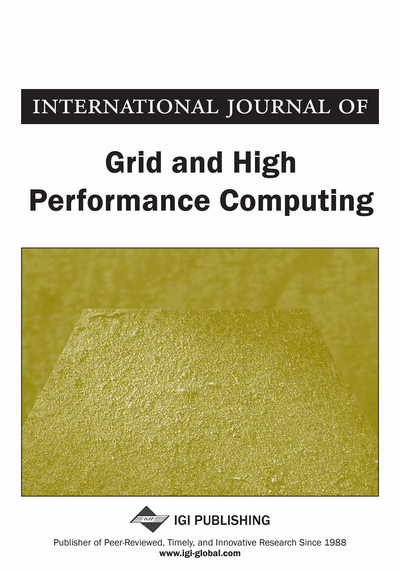# Statically Optimal Binary Search Tree Computation Using Non-Serial Polyadic Dynamic Programming on GPU's

DOI: 10.4018/IJGHPC.2019010104
OnDemand:
(Individual Articles)
Available
\$37.50
No Current Special Offers

## Abstract

Modern GPUs perform computation at a very high rate when compared to CPUs; as a result, they are increasingly used for general purpose parallel computation. Determining if a statically optimal binary search tree is an optimization problem to find the optimal arrangement of nodes in a binary search tree so that average search time is minimized. Knuth's modification to the dynamic programming algorithm improves the time complexity to O(n2). We develop a multiple GPU-based implementation of this algorithm using different approaches. Using suitable GPU implementation for a given workload provides a speedup of up to four times over other GPU based implementations. We are able to achieve a speedup factor of 409 on older GTX 570 and a speedup factor of 745 is achieved on a more modern GTX 1060 when compared to a conventional single threaded CPU based implementation.
Article Preview
Top

## 2. Non-Serial Polyadic Dynamic Programming Algorithm For Constructing A Statically Optimal Binary Search Tree

Ordinarily, a binary search tree contains records that are retrieved according to the values of the keys. Our goal is to organize the keys in a binary search tree so that the average time it takes to locate a key is minimized. A tree that is organized in this fashion is called optimal. A tree in which all the keys have an equal probability of being the search key is said to be balanced if the difference between the height of left and right sub-trees is kept to a minimum e.g. 1 or 2. However, we are concerned with the case where the keys do not have the same probability.

The number of comparisons done by the searching procedure to locate a key is called the search time. We will determine a tree for which the average search time is minimal. Let Key1, Key2… Keyn be the n keys in order, and let Pi be the probability that Keyi is the search key. If Ci is the number of comparisons needed to find Keyi in a given tree, the average search time for that tree is. Once the tree is constructed it cannot be modified hence the term statically optimal binary search tree.

Average Search Time = ∑ (Ci.Pi) where 1 ≤ i ≤ n (a)

This value is to be minimized. We can generalize this for i ≤ k ≤ j instead of only for 1 ≤ i ≤ n.

The Dynamic programming algorithm for the solution of this problem proceeds as follows. Let Ck be the number of comparisons needed to locate Keyk in the tree. We will call such a tree optimal for those keys and denote the optimal value by A[i][j]. Because it takes one comparison to locate a key in a tree which contains a single key hence; A [i][i] = Pi.

## Complete Article List

Search this Journal:
Reset
Volume 15: 2 Issues (2023)
Volume 14: 6 Issues (2022): 1 Released, 5 Forthcoming
Volume 13: 4 Issues (2021)
Volume 12: 4 Issues (2020)
Volume 11: 4 Issues (2019)
Volume 10: 4 Issues (2018)
Volume 9: 4 Issues (2017)
Volume 8: 4 Issues (2016)
Volume 7: 4 Issues (2015)
Volume 6: 4 Issues (2014)
Volume 5: 4 Issues (2013)
Volume 4: 4 Issues (2012)
Volume 3: 4 Issues (2011)
Volume 2: 4 Issues (2010)
Volume 1: 4 Issues (2009)
View Complete Journal Contents Listing